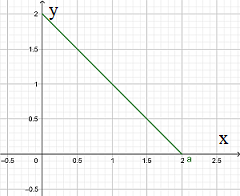# Describe and plot the curve given by parametric description. r(t) = t_x (2 cos^2 t) + t_y (2...

## Question:

Describe and plot the curve given by parametric description.

{eq}r(t) = t_x (2 \cos^2 t) + t_y (2 \sin^2 t) {/eq}

## Parametric Curve:

Let us consider a parametric curve in the plane described by the vector function

{eq}\vec r(t) = x(t) \hat t_x + y(t) \hat t_y {/eq}

where t is the parameter.

A sketch of the curve can be produced by plotting the sequence of points (x,y) as the paramter t varies

within a given interval {eq}[t_0,t_1]. {/eq}

The parametric curve specified by the vector function

{eq}\vec r(t) = 2 \cos^2 (t) \;\hat t_x + 2 \sin^2 (t) \; \hat t_y {/eq}

is the line with equation

{eq}x+y = 2\cos^2 t + 2\sin^2 t = 2 \Rightarrow y = 2-x. {/eq}

A sketch of the curve when t varies in the interval {eq}[0, 2\pi] {/eq}

is shown in the figure below.